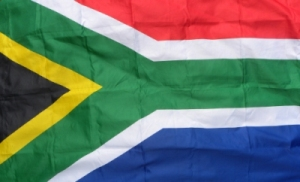# Cost of Goods Sold andInterest Expense

by Kim
(South Africa)(Please note that in the following question R = Rands, which is the South African currency)

a) Sales totaled R15,000,000
b) Cash R370,000
c) Marketable securities R259,000
d) Cash equivalents R250,900
e) Operating profit margin 35%
f) Operating expenses R3,250,000
g) Inventory turnover 6.0
h) There are 365 days in a year
i) The average collection period was 40 days
j) The current ratio is 1.75
k) The total asset turnover 1
l) The debt ratio is 60%
m) Net profit margin 8%
n) Return on equity 20%
o) Tax rate 40%
p) Notes payable R25,000
q) Retained earnings R60,000

CAN ANYONE PLS ANSWER WHAT IS THE AMOUNT FOR COST OF GOODS SOLD AND THE INTEREST EXPENSE?

### Comments for Cost of Goods Sold andInterest Expense

 my guess by: Anonymous my calculation is 2.5 million please double checkand would appreciate hearing from you.if you have the answer send to hijaziosama-atyahoo.comThanks